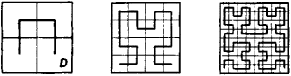# Peano Curve

## Peano curve

[pā′än·ō ‚kərv]
(mathematics)
A continuous curve that passes through each point of the unit square.
McGraw-Hill Dictionary of Scientific & Technical Terms, 6E, Copyright © 2003 by The McGraw-Hill Companies, Inc.
The following article is from The Great Soviet Encyclopedia (1979). It might be outdated or ideologically biased.

## Peano Curve

a continuous curve in the Jordan sense that entirely fills a square—that is, the curve passes through all the points of the square. The first example of a curve possessing thisFigure 1

property was constructed by G. Peano in 1890, and a simple example of a Peano curve was given by D. Hilbert in 1891. The initial steps of Hilbert’s construction are illustrated in Figure 1.

The limiting curve obtained by continuing the construction ad infinitum will be a Peano curve that passes through all the points of the square D.

Mentioned in ?
References in periodicals archive ?
Figure 9a shows the Peano curve. Figure 9b shows [X.sub.scale] of Peano curve using the CM partition.
We found that Peano curve is self-similar, Moore curve is not self-similar, Meander curve is self similar and Lebesgue curve is self-similar.
Both references use the existence of a continuous surjection from [0, 1] onto [[0, 1].sup.2] (a Peano curve in [[0, 1].sup.2] or a spacefilling curve).
Considering that the dynamics of the complex system entities take place on continuous but nondifferentiable curves, that is, fractal curves (e.g., the Koch curve, the Peano curve, or the Weierstrass curve [11-14,17,18]), they are given by the fractal operator [??]/dt (for details see Appendices A and B):
Having in view the geometrical characteristics, the Peano curve fractal may be used for heat pipe device.
For example a Peano curve is built by Hilbert (Fig.
In this example, we will look on the Peano curve and show how we can construct a Chinese lattice based on this curve.
Let us recall the shape and properties of the Peano curve. There are many ways of defining a Peano curve.
This would enable the construction of other forms of space-filling curve, such as the Peano curve.
All well-known fractal curves, such as Koch curve , Peano curve , Giuseppe Peano curve , and Hilbert Curve [23, 24], are preferably designed into dipole or monopole antennas.
The results from the Section Peano Curves and Semigroup ability were a part of a very first version of the paper .
Because Giuseppe Peano (1858-1932) was the first to discover one of the filling curve constructions, space-filling curves in 2-dimensional planes are sometimes called Peano curves. Some of the most celebrated are the Hilbert curve and the Sierpimki curve .

Site: Follow: Share:
Open / Close